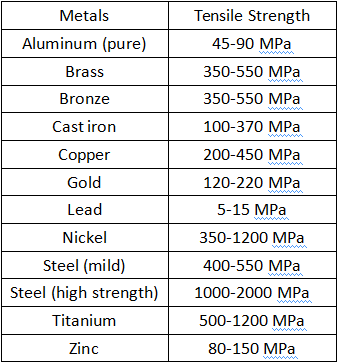> > What is Tensile Strength & What Determines It | Metal Tensile Strength Chart and Unit
> > What is Tensile Strength & What Determines It | Metal Tensile Strength Chart and Unit

# What is Tensile Strength & What Determines It | Metal Tensile Strength Chart and Unit

2023.2.22

When selecting the best material for making the final machined parts, it is important to consider the properties of the metals or plastics, one of which is tensile strength. So what is metal tensile strength and what determines it?

## What is Tensile Strength for Metals and Materials?

Tensile strength is a property of materials that measure their ability to resist deformation or failure under tensile or stretching forces. For metals, tensile strength is the maximum amount of tensile stress a material can withstand before it fractures or breaks. Tensile strength is an important property for many applications, particularly in structural and mechanical engineering. It is often used to determine the load-bearing capacity of a material or to design components that must withstand high stress or tension.

## Metal Tensile Strength Units - MPa to PSI

The tensile strength can be expressed in different units depending on the region or application. The most common units for tensile strength are megapascals (MPa) and pounds per square inch (psi).

MPa is the unit of pressure in the International System of Units (SI). 1 MPa is equivalent to 145 psi. Therefore, 1 psi is equal to approximately 0.00689 MPa. For example, if a material has a tensile strength of 100 MPa, it can be converted to 14,500 psi. Conversely, if a material has a tensile strength of 10,000 psi, it is equivalent to approximately 68.95 MPa. While both units are commonly used to express tensile strength, MPa is more commonly used internationally, particularly in scientific and engineering applications, whereas psi is more commonly used in the United States.

## What Determines Tensile Strength?

The tensile strength can depend on a variety of factors. Some of the key factors that affect tensile strength include:

– Material composition: The chemical composition of a material can have a significant impact on its tensile strength. For example, the addition of certain alloying elements can strengthen the material and improve its tensile strength.

– Manufacturing process: The way a material is produced can also affect its tensile strength. Factors such as temperature, pressure, and cooling rate can all impact the material’s internal structure and, as a result, its strength.

– Grain size: The size of the grains or crystals in a material can also impact its tensile strength. In general, materials with smaller grains tend to have higher tensile strength.

– Temperature: The temperature at which a material is used can impact its tensile strength. In some cases, materials may become brittle at low temperatures, reducing their ability to resist tensile stresses.

– Environmental conditions: The environment in which a material is used can also affect its tensile strength. For example, exposure to moisture, chemicals, or radiation can all degrade a material’s strength over time.

– Loading direction: The direction of the applied stress can also impact a material’s tensile strength. In some cases, materials may be stronger in one direction than in another due to their internal structure.

## Does High Tensile Strength Mean Strong?

Tensile strength is one of the factors that determine the overall strength of a material, but it is not the only factor. The strength of a material is a complex property that depends on many factors, including its tensile strength, its hardness, its ductility, its toughness, and its resistance to other forms of stress such as compression, bending, or torsion.

So, a material with high tensile strength does not necessarily mean that it is strong overall. For example, a brittle material with high tensile strength may be prone to sudden fracture or failure when subjected to impact or other forms of stress, whereas a tough and ductile material with moderate tensile strength may be better able to absorb energy and resist deformation under a wider range of stress conditions.

## Metal Tensile Strength Chart

Tensile strength and yield strength are important properties of aluminum and other metals. Here are the tensile strength ranges of some common metals, including aluminum, brass, steel, titanium, etc., these values are approximate and can vary depending on the specific composition, manufacturing process, and testing conditions.